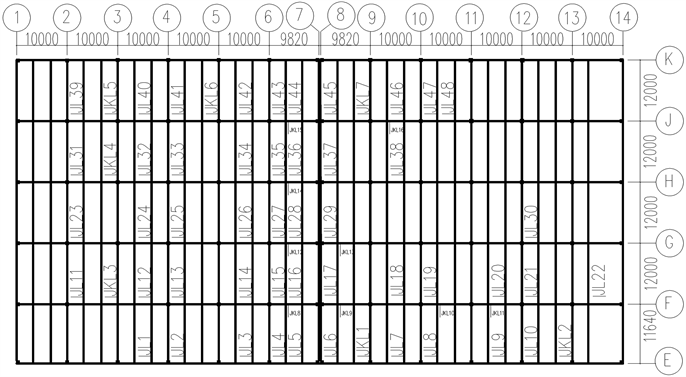#### 期刊菜单

The Calculation Method of Using High-Efficiency Prestressing Method to Strengthen Beams Reinforced with CFRP Sheets
DOI: 10.12677/HJCE.2022.115083, PDF, HTML, XML, 下载: 111  浏览: 163

Abstract: Based on the original design with pasting carbon fiber reinforcement beam due to special reasons to switch to efficient prestressed reinforcement method of engineering examples, based on the unification of the bearing capacity of normal section flexural member reinforced calculation formula method, the explicit expression and through the analysis, and puts forward the pasting CFRP reinforcement to efficient prestressed reinforcement calculation method. The applicability of the proposed method is illustrated by examples.

1. 引言Figure 1. Plan of the third floor

2. 加固计算公式推导Figure 2. Calculation diagram of bearing capacity

${H}_{op}=c+\eta \left(h+{a}_{p}-c\right)-{x}_{0}$ (1)

${x}_{p}={H}_{op}-\sqrt{{H}_{op}^{2}-\frac{2\Delta M}{{\alpha }_{1}{f}_{c}b}}$ (2)

${N}_{t}={\alpha }_{1}{f}_{c}b{x}_{p}$ (3)

${A}^{*}=N/\left[\sigma \right]$ (4)

$h$ ——原梁高度；

${a}_{p}$ ——加固材料受力合力点到原梁受拉边缘的距离；

${x}_{0}$ ——原梁计算受压区高度。

2.1. 采用粘贴CFRP布加固

$\left[\sigma \right]={\psi }_{f}{f}_{f}$ (5a)

${\psi }_{f}=\left(0.8{\epsilon }_{cu}h/{x}_{0}-{\epsilon }_{cu}-{\epsilon }_{{f}_{0}}\right)/{\epsilon }_{f}$ (5b)

${f}_{f}$ ——CFRP布抗拉强度设计值；

${\epsilon }_{cu}$ ——混凝土极限压应变，取为0.0033；

${\epsilon }_{f0}$ ——碳纤维复合材料拉应变设计值。

2.2. 采用高效预应力方法加固

${\sigma }_{pu}={\sigma }_{con}-\sum {\sigma }_{pi}+\Delta \sigma$ (6)

${\sigma }_{con}=0.55{f}_{ptk},\text{\hspace{0.17em}}\sum {\sigma }_{pi}=200\text{\hspace{0.17em}}\text{MPa},\text{\hspace{0.17em}}\Delta \sigma =50\text{\hspace{0.17em}}\text{MPa}$

$\left[\sigma \right]=0.55{f}_{ptk}-150$ (7)

2.3. 将粘贴CFRP布加固改高效预应力加固

1) 计算被加固梁的ΔM

$\Delta M=\left(2{x}_{p}{H}_{op}-{x}_{p}^{2}\right)\frac{{\alpha }_{1}{f}_{c}b}{2}$ (8a)

${H}_{op}=h-{x}_{0}$ (8b)

${x}_{p}={\psi }_{f}{f}_{f}{A}_{f}/\left({\alpha }_{1}{f}_{c}b\right)$ (8c)

2) 计算改用高效预应力方法加固时，预应力钢绞线面积 ${A}_{p}$

${H}_{op}=1.025h+1.05{a}_{p}-{x}_{0}$ (9)

${A}_{P}={\alpha }_{1}{f}_{c}b{x}_{p}/\left(0.55{f}_{ptk}-150\right)$ (10)

3) 计算钢绞线根数n：

$n=\left\{\begin{array}{l}{A}_{p}/140选用{\Phi }^{s}15.2钢绞线时\hfill \\ {A}_{p}/98.7选用{\Phi }^{s}12.7钢绞线时\hfill \end{array}$ (11)

3. 算例(以③轴线F-G梁F支座负弯矩加固为例)

3.1. 原梁参数

3.2. 反算ΔM

1) 计算原梁 ${x}_{0}$

${x}_{0}=\left({f}_{y}{A}_{s}-{{f}^{\prime }}_{y}{{A}^{\prime }}_{s}\right)/\left(\alpha {f}_{c}b\right)=360×\left(4418-2724\right)/\left(16.7×300\right)=121.73\text{\hspace{0.17em}}\text{mm}$

2) 计算原碳纤维加固的等效拉力 ${N}_{f}$

${\psi }_{f}=\left(0.8{\epsilon }_{cu}h/{x}_{0}-{\epsilon }_{cu}-{\epsilon }_{f0}\right)/{\epsilon }_{f}=\left[\left(\frac{0.8×0.0033×0.95}{0.12173}\right)-0.0033\right]/0.01=1.73$

$当{\psi }_{f}>1.0\text{\hspace{0.17em}}时，取{\psi }_{f}=1.0$

${A}_{f}=2×350×0.167=116.9\text{\hspace{0.17em}}{\text{mm}}^{2}$

${N}_{f}={\psi }_{f}{f}_{f}{A}_{f}=1.0×116.9×1600=163.66\text{\hspace{0.17em}}\text{kN}$

3) 计算粘贴碳纤维布加固时 ${H}_{op},{x}_{p}$

${H}_{op}=h-{x}_{0}=950-121.73=828.27\text{\hspace{0.17em}}\text{mm}$

${x}_{p}={N}_{f}/\left({\alpha }_{1}{f}_{c}b\right)=163.66×{10}^{3}/\left(16.7×300\right)=32.67\text{\hspace{0.17em}}\text{mm}$

4) 计算ΔM

$\Delta M=\left(2{x}_{p}{H}_{op}-{x}_{p}^{2}\right)\frac{{\alpha }_{1}{f}_{c}b}{2}=\left(2×32.67×828.27-{32.67}^{2}\right)×\frac{16.7×300}{2}=132.90\text{\hspace{0.17em}}\text{(kN}\text{ }\cdot \text{ }\text{m)}$

3.3. 高效预应力加固计算

1) 用式(9)计算高效预应力加固法的 ${H}_{op}$

${H}_{op}=1.025h+1.05{a}_{p}-{x}_{0}=1.025×950+1.05×45-121.73=899.27\text{\hspace{0.17em}}\text{mm}$

2) 用式(2)计算 ${x}_{p}$

${x}_{p}={H}_{op}-\sqrt{{H}_{op}^{2}-\frac{2\Delta M}{{\alpha }_{1}{f}_{c}b}}=899.27-\sqrt{{899.27}^{2}-\frac{2×132.90×{10}^{6}}{16.7×300}}=30.00\text{\hspace{0.17em}}\text{mm}$

3) 用式(10)计算高效预应力加固法的 ${A}_{p}$

${A}_{P}={\alpha }_{1}{f}_{c}b{x}_{p}/\left(0.55{f}_{ptk}-150\right)=\left(16.7×300×30.00\right)/\left(0.55×1860-150\right)=172.17\text{\hspace{0.17em}}\text{mm}$

4) 代入公式(11)求得高效预应力加固法钢绞线面积 ${A}_{p}$

① 选用Φs 15.2钢绞线时， $n={A}_{p}/140=172.17/140=1.2\approx 2根$

② 选用Φs 12.7钢绞线时， $n={A}_{p}/98.7=172.17/98.7=1.74\approx 2根$

4. 结语

NOTES

*通讯作者。

  吕恒林, 李延和, 李海涛, 等. 土木工程结构检测鉴定与加固改造[M]. 北京: 中国建筑工业出版社, 2019.  李延和, 陈贵, 李树林, 等. 高效预应力加固理论及应用[M]. 北京: 科学出版社, 2008.  施明峰. 不同参数下预应力钢绞线加固RC梁疲劳性能和破坏机制研究[D]: [硕士学位论文]. 泉州: 华侨大学, 2019.  张裕东, 唐雍巍, 马秀芬. 混凝土框架梁FRP加固施工技术研究[J]. 河南科技, 2020(14): 110-112.  裔博, 李延和, 任生元. 钢筋混凝土受弯构件加固计算的统一公式法[C]//中国基本建设优化研究会. 第十八届全国现代结构工程与环境优化技术交流会论文集. 北京: 知识产权出版社, 2012: 274-279.